## Classification

#### Classification

Direction: Select the one which is different from the other three responses.

1. '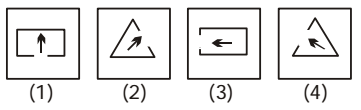1. In all other figures the arrow points towards open side.

##### Correct Option: A

Except in figure (1) , all other figures the arrow points towards open side.

1. '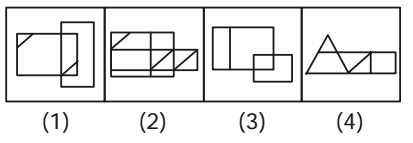1. In all other figures there are two intersecting quadrilaterals.

##### Correct Option: D

Except in figure (4), in all other figures there are two intersecting quadrilaterals.

1. '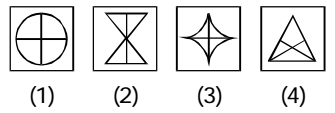1. Except one figure ,all others have been divided into four equal parts.

##### Correct Option: D

Except figure (4) all others have been divided into four equal parts.

1. '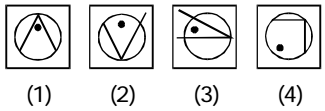1. As we can see that all other figures the two line segments form an acute angle.

##### Correct Option: D

As we can see that
Except figure (4), in all other figures the two line segments form an acute angle.

1. '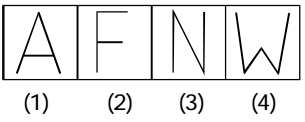1. All other designs have been made of three line segments .

##### Correct Option: D

All other designs have been made of three line segments whereas figure (4) has been made up of four line segments.